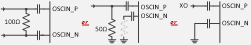If you have a related question, please click the "Ask a related question" button in the top right corner. The newly created question will be automatically linked to this question.

# LMX2820: SINGLE-ENDED CMOS CLOCK INPUT

Part Number: LMX2820

Is it okay to not need a 50Ω resistor on the OSCIN_P pin when inputting a CMOS clock to the OSCIN_P pin? Is it okay to input a 50MHz CMOS clock with high impedance?

Reference Does the phase noise performance change depending on the input level of Oscillator Input? I want to check if the phase noise is dependent on the Reference level.

・Data sheet Figure 6-8. For Output Power vs. OUTx_PWR, is there a regulation for each power step of OUTx_PWR 0 to 7? (If the OUTx_PWR value is changed by 1, it changes by 〇 dB, etc.) Figure 6-8. Shows three graphs of OUTx_PWR = 0,4,7, but the power difference between OUTx_PWR = 0 and 4 and the power difference between OUTx_PWR = 4 and 7 are significantly different, so check the above. I want to

• Hi Shimizu-san,

Yes, direct connection to an XO is possible.To get the best performance, (1) the reference clock input level as specified in the Electrical Characteristics section of the datasheet should be met. (2) phase noise of the reference clock should be as low as possible. (3) the slew rate of the reference clock should also be as high as possible.

The output power control is in fact a current control to the output buffer, as such, the output power change is not a precise linear increment.

• Hi Noel,

The data sheet stipulates that the OSCin input voltage (single-ended) is 0.3 to 3.6 Vpp.
Is there a change in the phase noise performance of the RF output between 0.3 and 3.6Vpp?
Is the higher OSCin input voltage better than the lower OSCin input voltage? Or is there no change in phase noise between 0.3 and 3.6 Vpp?

• Hi Shimizu-san,

That's depend on the frequency and waveform.

If you have a 10MHz sinewave reference clock, then the voltage swing will be matter. Higher voltage swing results in higher slew rate.

However, for a 100MHz sinewave clock, the voltage swing does not matter as the slew rate is already high.

If the input is a square wave clock, then frequency and voltage swing are not matter. The reason is very obvious, the slew rate is same for all frequencies and does not depend on voltage swing.